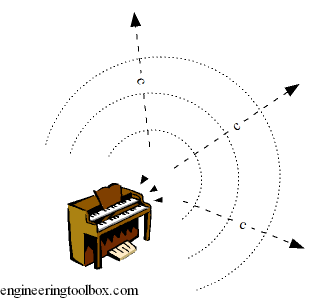Engineering ToolBox - Resources, Tools and Basic Information for Engineering and Design of Technical Applications!

# Speed of Sound Equations

## Calculate the speed of sound (the sonic velocity) in gases, fluids or solids.

A disturbance introduced in some point of a substance - solid or fluid - will propagate through the substance as a wave with a finite velocity.### Acoustic Velocity and Speed of Sound

The velocity at which a small disturbance will propagate through the medium is called Acoustic Velocity or Speed of Sound.

Note that speed is a scalar quantity. Velocity is vector quantity with direction.

The acoustic velocity is related to the change in pressure and density of the substance and can be expressed as

c = (dp / dρ)1/2                                  (1)

where

c = speed of sound (m/s, ft/s)

dp = change in pressure (Pa, psi)

dρ = change in density (kg/m3, lb/ft3)

### Speed of Sound in Gases, Fluids and Solids

The acoustic velocity can alternatively be expressed with Hook's Law as

c = (K / ρ)1/2                                 (2)

where

K = Bulk Modulus of Elasticity (Pa, psi)

ρ = density (kg/m3, lb/ft3)

This equation is valid for liquids, solids and gases. The sound travels faster through media with higher elasticity and/or lower density. If a medium is not compressible at all - incompressible - the speed of sound is infinite (c ≈ ∞).

SubstanceBulk Modulus Elasticity
- K
-
(109 N/m2)
Density
- ρ
-
(kg/m3)
Water 2.15 999.8
Oil 1.35 920
Ethyl Alcohol 1.06 810
Mercury 28.5 13595
• properties at 1 bar and 0 oC

### Speed of Sound - Sonic Velocity - in Ideal Gases

Since the acoustic disturbance introduced in a point is very small the heat transfer can be neglected and for gases assumed isentropic. For an isentropic process the ideal gas law can be used and the speed of sound can be expressed as

c = (k p / ρ)1/2

= (k R T)1/2                                               (3)

where

k = ratio of specific heats (adiabatic index)

p = pressure (Pa, psi)

R = individual gas constant (J/kg K, ft lb/slug oR)

T = absolute temperature (oK, oR)

For an ideal gas the speed of sound is proportional to the square root of the absolute temperature.

### Example - Speed of Sound in Air

The speed of sound in air at 0 oC (273.15 K) and absolute pressure 1 bar can be calculated as

c = (1.4 (286.9 J/K kg) (273.15 K))1/2

= 331.2 (m/s)

where

k = 1.4

and

R = 286.9 (J/K kg)

The speed of sound in air at 20 oC (293.15 K) and absolute pressure 1 bar can be calculated as

c = (1.4 (286.9  J/K kg) (293.15 K))1/2

= 343.1 (m/s)

### Example - Speed of Sound in Water

The speed of sound in water at 0 oC can be calculated as

c = ((2.06 109 N/m2) / (999.8 kg/m3))1/2

= 1435.4 (m/s)

where

Ev= 2.06 109 (N/m2)

and

ρ = 999.8 (kg/m3)

### Subsonic and Supersonic speed

• If the Mach Number is below 1, the flow velocity is lower than the speed of sound - and the speed is subsonic.
• If the Mach Number is 1 - the speed is transonic.
• If the Mach Number is above 1, the flow velocity is higher than the speed of sound - and the speed is supersonic.

## Related Topics

• Acoustics - Room acoustics and acoustic properties - decibel A, B and C - Noise Rating (NR) curves, sound transmission, sound pressure, sound intensity and sound attenuation
• Fluid Mechanics - The study of fluids - liquids and gases. Involves velocity, pressure, density and temperature as functions of space and time

## Engineering ToolBox - SketchUp Extension - Online 3D modeling!

Add standard and customized parametric components - like flange beams, lumbers, piping, stairs and more - to your Sketchup model with the Engineering ToolBox - SketchUp Extension - enabled for use with the amazing, fun and free SketchUp Make and SketchUp Pro .Add the Engineering ToolBox extension to your SketchUp from the SketchUp Pro Sketchup Extension Warehouse!

Translate

## Privacy

We don't collect information from our users. Only emails and answers are saved in our archive. Cookies are only used in the browser to improve user experience.

Some of our calculators and applications let you save application data to your local computer. These applications will - due to browser restrictions - send data between your browser and our server. We don't save this data.

## Citation

• Engineering ToolBox, (2003). Speed of Sound Equations. [online] Available at: https://www.engineeringtoolbox.com/speed-sound-d_82.html [Accessed Day Mo. Year].

Modify access date.

. .

#### Scientific Online Calculator10 15